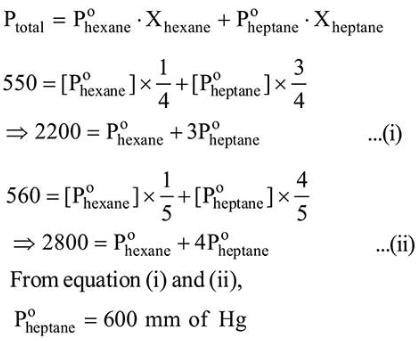# At 300 K, the vapour pressure of a solution containing 1 mole of n-hexane and 3 moles of $n$-heptane is 550 mm of Hg.Question:

At $300 \mathrm{~K}$, the vapour pressure of a solution containing 1 mole of $n$-hexane and 3 moles of $n$-heptane is $550 \mathrm{~mm}$ of Hg. At the same temperature, if one more mole of $n$ heptane is added to this solution, the vapour pressure of the solution increases by $10 \mathrm{~mm}$ of $\mathrm{Hg}$. What is the vapour pressure in $\mathrm{mm} \mathrm{Hg}$ of $n$-heptane in its pure state ________________

Solution:

(600)